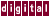## 7.3.5 Invariant If Floating

When the `if` condition is invariant in a loop, the `if` statement can be floated out of the assignment loop, as shown in the following example:

```for ( i=0; IPA; i++ ) {
a[i] = b[i] + c[i];
if ( x > 0.0 )
a[i] *= x;
}
```

Becomes:

```if (x > 0.0) {
for ( i = 0; i<n; i++ ) {
a[i] = b[i] + c[i];
a[i] *=  x;
}
} else {
for ( i = 0; i<n; i++ ) {
a[i] = b[i] + c[i];
}
}
```

See Chapter 4, the `/eiifg` and `/miifg` qualifiers, for information about controlling this transformation.

Previous Page Next Page Contents Index
Command-Line Qualifiers